## RS Aggarwal Class 7 Solutions Chapter 7 Linear Equations in One Variable CCE Test Paper

These Solutions are part of RS Aggarwal Solutions Class 7. Here we have given RS Aggarwal Solutions Class 7 Chapter 7 Linear Equations in One Variable CCE Test Paper.

Other Exercises

Question 1.
Solution:
We have:
x3 + y3 + z3 – 3xyz
= (-2)3 + (-1)3 + (3)3 – 3 x (-2) x (-1) x 3
= – 8 – 1 + 27 – 18 = -27 + 27 = 0

Question 2.
Solution:
Co-efficient of x in the given numbers are
(i) -5y
(ii) 2yz
(iii) $$\frac { -3 }{ 2 }$$ ab

Question 3.
Solution:
We have:
(4xy – 5x2 – y2 + 6) – (x2 – 2xy + 5y2 – 4)
= 4xy – 5x2 – y2 + 6 – x2 + 2xy – 5y2 + 4
= -6x2 – 6y2 + 6xy +10
= -2 (3x2 + 3y – 3xy – 5)

Question 4.
Solution:
We have:
(2x2 – 3y2 + xy) – (x2 – 2xy + 3y2)
= 2x2 – 3y2 +xy – x2 + 2xy – 3y2
= 2x2 – x2 – 3y2 – 3y2 + xy + 2xy
= x2 – 6y2 + 3xy
x2 – 2xy + 3y2 is less than 2x2 – 3y2 + xy by x2 – 6y + 3xy.

Question 5.
Solution:
We have: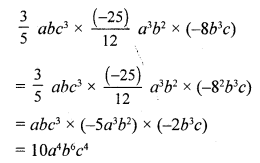Question 6.
Solution:
We have:
(3a + 4) (2a – 3) + (5a – 4) (a + 2)
= {3a (2a – 3) + 4 (2a – 3)} + {5a (a + 2) – 4 (a + 2)}
= (6a2 – 9a + 8a – 12) + (5a2 + 10a – 4a – 8)
= (6a2 – a – 12) + (5a2 + 6a – 8)
= (11a2 + 5a – 20)

Question 7.
Solution:Question 8.
Solution:
We have: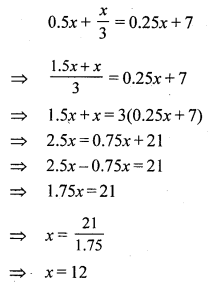Question 9.
Solution:
Let the consecutive odd number be
x and (x + 2)
x + (x + 2) = 68
2x + 2 = 68
2x = 68 – 2 = 66
x = 33
The required numbers are 33 and (33 + 2), i.e., 35.

Question 10.
Solution:
Let Reenu’s present age be x
Then, her father’s present age will be 3x
Reenu’s age after 12 years = (x + 12)
3x + 12 = 2x + 24
x = 12
Reenu’s present age = x = 12 yrs
And her father’s age = 3x = (3 x 12) = 36 yrs

Mark (✓) against the correct answer in each of the following :
Question 11.
Solution: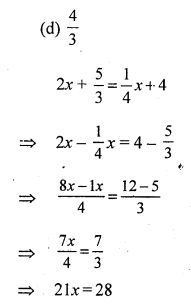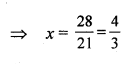Question 12.
Solution:Question 13.
Solution:Question 14.
Solution:
(c) 18
Let the number be x.
According to the equation, we have:
4x = x + 54
⇒ 3x = 54
⇒ x = 18

Question 15.
Solution:
(b) 52°
Let the two complementary angles be x° and (90 – x)°.
According to the equation, we have:
x – (90 – x) = 14
⇒ 2x = 104
⇒ x = 52
(90° – x)° = 90° – 52° = 38°
The larger angle is 52°.

Question 16.
Solution:
(c) 32 m
Let the length and breadth of the rectangle be l m and b m, respectively.
According to the questions, we have:
l = 2b ……(i)
2 (l + b) = 96 …..(ii)
Now, 2 (2b+ b) = 96
⇒ 6b = 96
⇒ b = 16
Length = 16 x 2 m = 32 m

Question 17.
Solution:
(b) 12 years
Let the ages of A and B be x and y years respectively,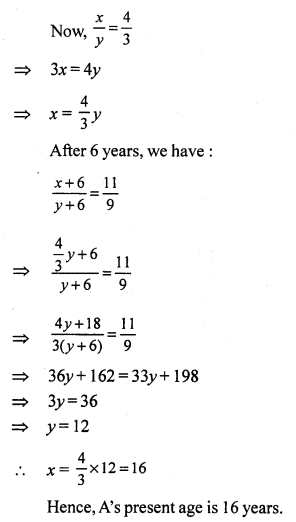Question 18.
Solution:
(i) -2a2 b is a monomial.
(ii) (a2 – 2b2) is a binomial.
(iii) (a + 2b – 3c) is a trinomial.
(iv) In -5ab, the coefficient of a is -5.
(v) In x2 + 2x – 5, the constant term is -5.

Question 19.
Solution:
(i) False.
The coefficient of x is -1.
(ii) False.
The coefficient of x in – 3.
(iii) False.
LHS = (5x – 7) – (3x – 5) = 5x – 7 – 3x + 5 = 2x – 2.
(iv) True.
LHS = (3x + 5y) (3x – 5y)
= 3x (3x – 5y) + 5y (3x – 5y)
= 9x2 – 15xy + 15xy – 25y2
= 9x2 – 25y2
(v) True
(a2 + b2)Hope given RS Aggarwal Solutions Class 7 Chapter 7 Linear Equations in One Variable CCE Test Paper are helpful to complete your math homework.

If you have any doubts, please comment below. Learn Insta try to provide online math tutoring for you.